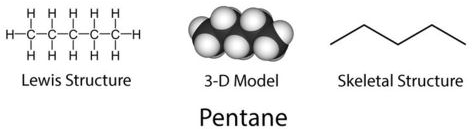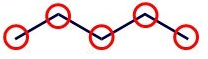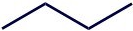# 4.2: Representing Structures

•• Contributed by Allison Soult
• Senior Lecturer (Chemistry) at University of Kentucky

Learning Outcomes

• Use condensed structural formulas to represent molecules.
• Use skeletal structures to represent molecules.

Lewis structures are great for small molecules but can be complicated when dealing with large molecules. There are a variety of ways that we can represent molecules that provide use with enough information without creating a complicated figure. The structures in the figure below all represent the molecules pentane, which is a straight-chain alkane with five carbon atoms.## Lewis Structures

The Lewis structure shows bonds to represent the sharing of electrons and include all atoms in the molecule so we have information about connectivity of atoms but not the molecular shape or geometry.

## Space-Filling Model

A 3-D space-filling model shows connectivity of atoms and the shape of the molecule. These work well for smaller molecules, but can be a challenge to interpret since atoms are so close together. This is the most accurate representation of what a molecule looks like.

## Skeletal Structure

These are the simplest way to represent the structure of a molecule. Hydrogen atoms are omitted (except when attached to $$\ce{O}$$ or $$\ce{S}$$) and carbon atoms are at the intersection of each pair of lines and at the terminal end of each line. Each of the circles in the figure below indicate where a carbon is located.Figure $$\PageIndex{1}$$: Pentane molecule with circles indicating locations of carbon atoms.

## Condensed Structures

These provide more information than a chemical formula (like $$\ce{C_5H_{12}}$$ for pentane) but are condensed as given by their name, so they can be written out rather than in a drawing. The best way to write a condensed structure is to "read" a Lewis structure and see how atoms are connected.

Example $$\PageIndex{1}$$

Draw the skeletal and condensed structure of 2-butanol based on the given Lewis structure.Skeletal Structure

Count the number of carbon atoms and draw the carbon chain with the correct number of carbon atoms. This represents a chain of four carbon atoms.Now look for any atoms other than hydrogen that are attached to the carbon chain. The second carbon has an $$\ce{-OH}$$ group. Add that to the second carbon in the chain.The skeletal structure is complete and all of the remaining hydrogen atoms are implied because every carbon must have four bonds.

Condensed Structure

For the condensed structure, we "read" the Lewis structure from left to right.

The first carbon has three hydrogen atoms attached so we start with

$\ce{CH_3}$

The second carbon has one hydrogen attached and one $$\ce{-OH}$$ group. Since the $$\ce{-OH}$$ group is to the side, we put it in parentheses

$\ce{CH_3CH(OH)}$

The third carbon has two hydrogen atoms attached so we add $$\ce{CH_2}$$

$\ce{CH_3CH(OH)CH_2}$

The fourth, and last, carbon has three hydrogen atoms attached, so we add $$\ce{CH_3}$$

$\ce{CH_3CH(OH)CH_2CH_3}$

The result is the condensed structure of 2-butanol.